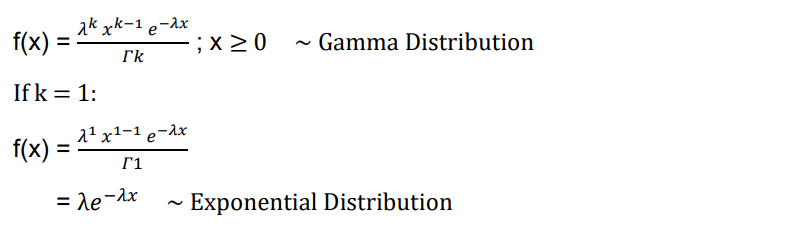## Introduction

In probability and statistics, the waiting time before the occurrence of an event is said to follow Exponential Distribution if the probability that the given event occurs during a certain time interval is proportional to the length of that time interval.

Exponential Distribution can calculate the time between events in a Poisson point process, wherein an event can occur continuously and independently at a constant average rate.

## Exponential Distribution

Exponential Distribution is one of the most widely used cumulative distributions. Exponential Distribution is concerned with the amount of time taken until a specific event occurs. For example, Exponential Distribution can be used to tell the amount of time a shopkeeper needs to wait until a customer arrives, or the time taken before a piece of machinery breaks down.Here:

• λ = 1/mean (λ > 0)
• x = random variable

The following are the properties of Exponential Distribution:

• Mean = 1/λ
• Variance = 1/(λ^2)

#### Particular Case of Gamma Distribution

The Exponential Distribution falls under a specific case of Gamma Distribution. The formula can be derived as follows:Here, k = number of times success occurs

In Exponential Distribution, since we are concerned with only the occurrence of the first successful event, we can take the value of k to be 1.

Let us assume that the mileage that certain car owners get with a certain kind of tire is a random variable having an Exponential Distribution with a mean of 40,000 km. The probability that one of these tires will last at most 30,000 km will take every value between 0 and 30,000. Therefore, we can integrate the formula with these limits, as shown below:#### Implementation

Let us try to code the example that we worked on above. We first create a function that computes the Exponential Distribution and then declare values to get the required output.

				
fun exponential(x1: Double, x2: Double, mean: Double): Double
{
return Math.exp(-x1 / mean) - Math.exp(-x2 / mean)
}



In this function, it can be observed that the function has been created in a way that implementation of integration will not be necessary. The final answer of the integration, along with the substitution of limits is enough to get an accurate answer, while optimizing the code at the same time.

				
val mean = 40000.0
val x1 = 0.0       //lower limit of integration
val x2 = 30000.0  //upper limit of integration
println(exponential(x1, x2, mean))



Output:

				
0.5276334472589853



Note: If the goal is to find the probability that a tire will last at least a certain distance instead of at most, then the code can be modified to find: 1 – exponential(x1, x2, mean)